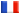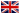# Séminaire de Cryptographie

## Benoit Chevallier-Mames### Linear Bandwidth Naccache-Stern Encryption

The Naccache-Stern (NS) knapsack cryptosystem is a little-known public-key encryption scheme, despite (or because of) its original design. In this scheme, the ciphertext is obtained by multiplying the public-keys indexed by the message bits modulo a prime p. The cleartext is then recovered by factoring the ciphertext raised to a secret power modulo p.

NS encryption requires a multiplication per two plaintext bits on the average, while decryption is roughly as costly as an RSA decryption. However, NS features a bandwidth sublinear in log(p), namely log(p)/log(log(p)).

This presentation presents new NS variants allowing to reach bandwidths linear in log(p). The price to pay for reaching a linear bandwidth is a public-key of size log3(p)/log(log(p)). Beyond their mathematical interest, these modifications can possibly make the NS knapsack cryptosystem more practical and attractive.

The presentation will be held in French, and will be self-included as much as possible.

This is a joint work with David Naccache (U. Paris II, ENS) and Jacques Stern (ENS) .